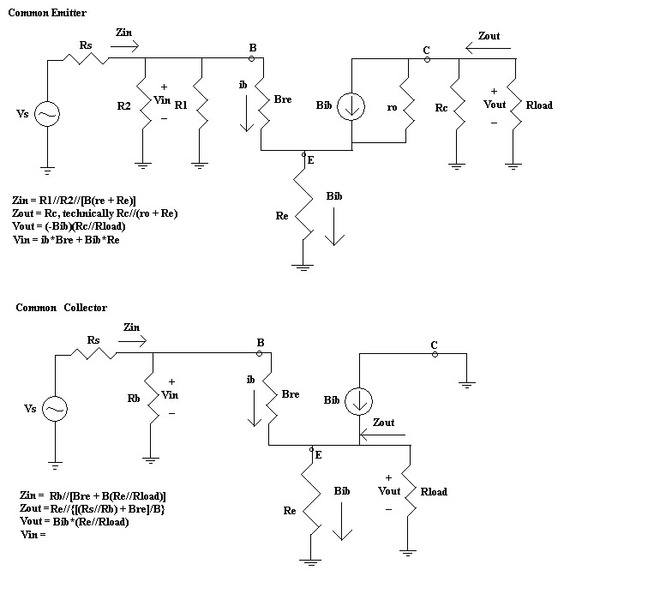# Transistor re AC Model

• Number2Pencil

#### Number2Pencil

This is more of a "help me understand" than "help me find the answer" problem. forgive me for not using the template.

this is the "little r-e" model, similar to the hybrid modelI'm having a bit of trouble understanding how my professor got the equations for these two examples.

\\\\\\\\\\\\\\\\\\\\\\\\\\\\\
Common Emitter:
/////////////////////////////

Zin: why does Re get multiplied by Beta?
Why does he not consider ro, Rc, or Rload?

Zout: Why does he not consider Bre, R1, R2, or Rs?

\\\\\\\\\\\\\\\\\\\\\\\\\\\\\
Common Collector:
/////////////////////////////

Zin: once again, Beta being multiplied by what is connected to the emitter?

Zout: now he DOES consider all the resistors on the left, AND divides it by Beta.

\\\\\\\\\\\\\\\\\\\\\\\\\\\\\
attempt
/////////////////////////////

I think I can see why Re is being multiplied by Beta in the common emitter. The current going through Bre is ib and the current going through Re is Bib, so apparently there needs to be a multiplying factor of Beta to make up for it...for what reason, I have no clue.

Last edited:
Common Emitter:

-Why does Re get multiplied by beta:

The answer is simple, what current goes through Re ? Ic, right? So Ic can be expressed as $$Ic = \beta\cdot I_{b}$$
So you'll get something like this:

$$V_{b} = I_{b}\cdot \beta r{e} + I_{c}\cdot R_{e}$$

substituting $$I_{c} = \beta I_{b}$$ gives:

$$V_{b} = I_{b}\cdot \beta r{e} + \beta \cdot I_{b}\cdot R_{e}$$

If you go a little be further, you can easily show that the gain of the amplifier doesn't depend on $$\beta$$
it only depends on $$\frac{R_{c}||R_{load}}{r_{e}+R_{e}}$$, since $$\beta I_{b}$$ gets canceled out.

Including $$r_{o}$$ into your calculation does not affect gain formula, since you'll get $$R_{c}||R_{load}||r_{o}$$ and as you know, combining very large impedance with small impedance, will result in small impedance, $$r_{o}||R_{c}||R_{load}$$ will be approximated to $$R_{c}||R_{load}$$

-Why does he not consider ro, Rc, Rload

ro is VERY large, hence it does not affect the circuit(open circuit), hence no point of using it.
Rc, Rload has nothing to do with the input impedance. Rc, Rload only affects output impedance.

Common collector:

Zin: same answer as before, rewrite Ic as combination of $$\beta$$ and Ib, you'll get the same answer.

Zout: Now there is no current source in front of the output resistors, hence all of the resistors have to be considered.

Tips: Read up on circuit theory, deriving equations for those circuits is essential!

Last edited:
hmm...so there is just an invisible dividing line that says "input impedance ends here"?

other than that, thanks I got it

For a 4-terminal network, Zin is defined as the input impedance when the output current is 0.

Zout is defined as the output impedance when the input current is 0.

That's where the "invisible divding line" comes from.

http://en.wikipedia.org/wiki/Two-port_network

Last edited:
"Invisible dividing line" - I like it ;) AlephZero is correct.